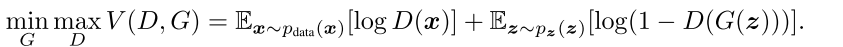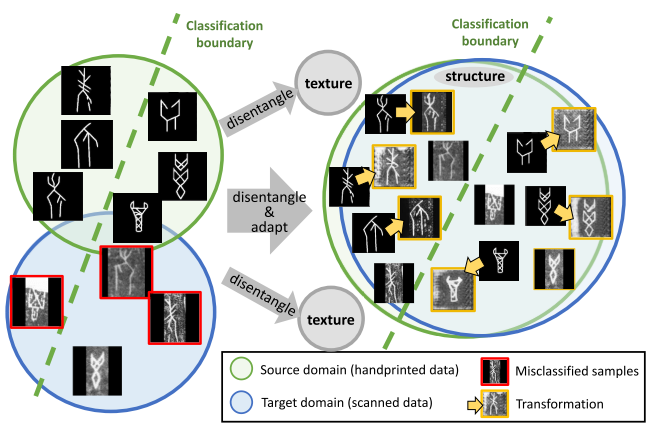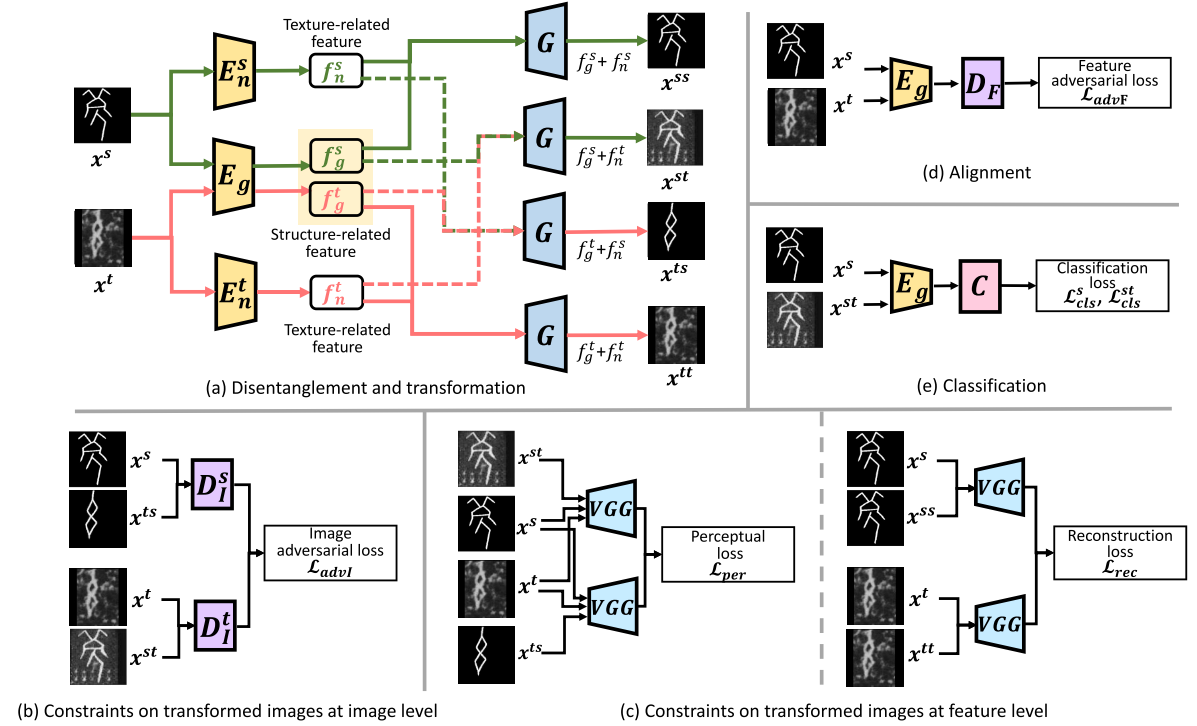# Introduction of generative adversarial networks (GANs)

Last updated on：2 years ago

GANs are widely used in generating new data such as images and sequences by using the training dataset. It can help us enlarge the dataset to balance data for deep learning jobs. The application seems like two stages of deep learning architecture.# Concepts

Understand the difference between generative and discriminative models.

## Generative

Generative models can generate new data instances and capture the joint probability $p(X, Y)$, or just $p(X)$ if there are no labels.

## Discriminative

Discriminative models discriminate between different data instances and capture the conditional probability $p(Y|X)$.

## GAN Training

Because a GAN contains two separately trained networks, its training algorithm must address two complications

GANs must juggle two different kinds of training (generator and discriminator).

GAN convergence is hard to identify.

Alternating Training

1. The discriminator trains for one or more epochs.
2. The generator trains for one or more epochs.
3. Repeat steps 1 and 2 to continue to train the generator and discriminator networks.

We keep the generator constant during the discriminator training phase. As discriminator training tries to figure out how to distinguish real data from fake, it has to learn how to recognize the generator’s flaws.

Similarly, we keep the discriminator constant during the generator training phase. Otherwise, the generator would be trying to hit a moving target and might never converge.

## Convergence

As the generator improves with training, the discriminator performance worsens because the discriminator can’t quickly tell the difference between real and fake. If the generator succeeds perfectly, then the discriminator has a $50%$ accuracy.

Mode Collapse

If a generator produces an especially plausible output, the generator may learn to produce only that output. The generator is always trying to find the one output that seems most reasonable to the discriminator.

Attempts to Remedy

Try to force the generator to broaden its scope by preventing it from optimizing.

During generator training, gradients propagate through the discriminator network to the generator network (although the discriminator does not update its weights). So the weights in the discriminator network influence the updates to the generator network.

While a GAN can use the same loss for both generator and discriminator training (or the same loss differing only in sign), it’s not required. In fact, it’s more common to use different losses for the discriminator and the generator.

## Loss function

The generator and discriminator losses look different in the end, even though they derive from a single formula.

Loss function:

$$\mathcal{L} = E_x [\log (D(x))] + E_z [\log (1 - D(G(z)))]$$

Conditional GANs loss function:## Q&A

1.Identify problems that GANs can solve.

Example uses

Create training data

Increase image resolution

Morph audio

2.Understand the roles of the generator and discriminator in a GAN system.

The discriminator in a GAN is simply a classifier. It tries to distinguish real data from the data created by the generator. It could use any network architecture appropriate to the type of data it’s classifying.

The generator part of a GAN learns to create fake data by incorporating feedback from the discriminator. It learns to make the discriminator classify its output as real.

GANs try to replicate a probability distribution. Therefore, they should use loss functions that reflect the distance between the distribution of the data generated by the GAN and the real data distribution.

Benefiting from disentanglement, transformation and alignment, handprinted and scanned data are aligned in structure-shared space and the network is optimized by transformed characters, which improve performance on scanned data.$E_g$, $E_n^s$ and $E_n^t$ encode images into structure and texture feature space. Based on the disentangled features, $G$ generates the reconstructed images.

$D^s_I$ and $D^t_I$ are utilized to make the transformed images look real from image level.

VGGNet ensures the generated images contain proper structures and textures at feature level.

$D_F$ is pitted against $E_g$ to make structure related features domain-variant.

$C$ stacked on $E_g$ is trained on the source and transformed target-like images which further improves the generalization and discrimination of network.Partial codes:

Encoder has average global pooling:

class SharedEncoder(nn.Module):
def __init__(self, resnet_name):
super(SharedEncoder, self).__init__()
model_resnet = resnet_dict[resnet_name](pretrained=True)
class_num = 241
self.conv1 = model_resnet.conv1
self.bn1 = model_resnet.bn1
self.relu = model_resnet.relu
self.maxpool = model_resnet.maxpool
self.layer1 = model_resnet.layer1
self.layer2 = model_resnet.layer2
self.layer3 = model_resnet.layer3
self.layer4 = model_resnet.layer4
self.avgpool = model_resnet.avgpool
self.layer0 = nn.Sequential(self.conv1, self.bn1, self.relu, self.maxpool)
self.feature_layers = nn.Sequential(self.layer1, self.layer2, self.layer3, self.layer4)
self.fc = nn.Linear(model_resnet.fc.in_features, class_num)
self.fc.apply(init_weights)
self.__in_features = model_resnet.fc.in_features
def forward(self, x):
low = self.layer0(x)
rec = self.feature_layers(low)
y = self.avgpool(rec)
y = y.view(y.size(0), -1)
y1 = self.fc(y)
return low, rec, y, y1

Inner backbone encoder:

class PrivateEncoder(nn.Module):
def __init__(self, input_channels, code_size):
super(PrivateEncoder, self).__init__()
self.input_channels = input_channels
self.code_size = code_size
self.cnn = nn.Sequential(nn.Conv2d(self.input_channels, 64, 7, stride=2, padding=3),
nn.BatchNorm2d(64),
nn.ReLU(),
nn.BatchNorm2d(128),
nn.ReLU(),
nn.BatchNorm2d(256),
nn.ReLU(),
nn.BatchNorm2d(256),
nn.ReLU(),
nn.BatchNorm2d(256),
nn.ReLU())
self.model = []
self.model += [self.cnn]
self.model += [nn.Conv2d(256, code_size, 1, 1, 0)]
self.model = nn.Sequential(*self.model)

def forward(self, x):
bs = x.size(0)
output = self.model(x).view(bs, -1)
return output

Decoder:

class PrivateDecoder(nn.Module):
def __init__(self, shared_code_channel, private_code_size):
super(PrivateDecoder, self).__init__()
num_att = 256
self.shared_code_channel = shared_code_channel
self.private_code_size = private_code_size
self.main = []
self.upsample = nn.Sequential(
nn.ConvTranspose2d(256, 256, 4, 2, 1, bias=False),
nn.InstanceNorm2d(256),
nn.ReLU(True),
Conv2dBlock(256, 128, 3, 1, 1, norm='ln', activation='relu', pad_type='zero'),
nn.ConvTranspose2d(128, 128, 4, 2, 1, bias=False),
nn.InstanceNorm2d(128),
nn.ReLU(True),
Conv2dBlock(128, 64 , 3, 1, 1, norm='ln', activation='relu', pad_type='zero'),
nn.ConvTranspose2d(64, 64, 4, 2, 1, bias=False),
nn.InstanceNorm2d(64),
nn.ReLU(True),
Conv2dBlock(64 , 32 , 3, 1, 1, norm='ln', activation='relu', pad_type='zero'),
nn.ConvTranspose2d(32, 32, 4, 2, 1, bias=False),       #add    # 56*56
nn.InstanceNorm2d(32),
nn.ReLU(True),
Conv2dBlock(32 , 32 , 3, 1, 1, norm='ln', activation='relu', pad_type='zero'),
nn.ConvTranspose2d(32, 32, 4, 2, 1, bias=False),        #add   # 112*112
nn.InstanceNorm2d(32),
nn.ReLU(True),
Conv2dBlock(32 , 32 , 3, 1, 1, norm='ln', activation='relu', pad_type='zero'),
nn.Conv2d(32, 3, 3, 1, 1),
nn.Tanh())
self.main += [ResBlocks(3, 256, 'ln', 'relu', pad_type='zero')]
self.main += [self.upsample]
self.main = nn.Sequential(*self.main)
self.mlp_att   = nn.Sequential(nn.Linear(private_code_size, private_code_size),
nn.ReLU(),
nn.Linear(private_code_size, private_code_size),
nn.ReLU(),
nn.Linear(private_code_size, private_code_size),
nn.ReLU(),
nn.Linear(private_code_size, num_att))
def forward(self, shared_code, private_code, d):
d = Variable(torch.FloatTensor(shared_code.shape, 1).fill_(d)).cuda()
d = d.unsqueeze(1)
d_img = d.view(d.size(0), d.size(1), 1, 1).expand(d.size(0), d.size(1), shared_code.size(2), shared_code.size(3))
att_params = self.mlp_att(private_code)
att_img = att_params.view(att_params.size(0), att_params.size(1), 1, 1).expand(att_params.size(0), att_params.size(1), shared_code.size(2), shared_code.size(3))
code = torch.cat([shared_code, att_img, d_img], 1)
output = self.main(code)
return output


# Reference

 Karras, T., Aila, T., Laine, S. and Lehtinen, J., 2017. Progressive growing of gans for improved quality, stability, and variation. arXiv preprint arXiv:1710.10196.

 Karras, T., Aittala, M., Laine, S., Härkönen, E., Hellsten, J., Lehtinen, J. and Aila, T., 2021, May. Alias-free generative adversarial networks. In Thirty-Fifth Conference on Neural Information Processing Systems.

 Gui, J., Sun, Z., Wen, Y., Tao, D. and Ye, J., 2020. A review on generative adversarial networks: Algorithms, theory, and applications. arXiv preprint arXiv:2001.06937.

 Wang, M., Deng, W. and Liu, C.L., 2022. Unsupervised Structure-Texture Separation Network for Oracle Character Recognition. IEEE Transactions on Image Processing.

 wm-bupt/STSN